Homework Help Question & Answers

# Suppose that the hatch on the side of a Mars lander is built and tested on...

Suppose that the hatch on the side of a Mars lander is built and tested on Earth so that the internal pressure just balances the external pressure. The hatch is a disk 50.0 cm in diameter. When the lander goes to Mars, where the external pressure is 650 N/m2, what will be the net force (in newtons and pounds) on the hatch, assuming that the internal pressure is the same in both cases? Will it be an inward or an outward force?

#### Homework Answers

Answer #1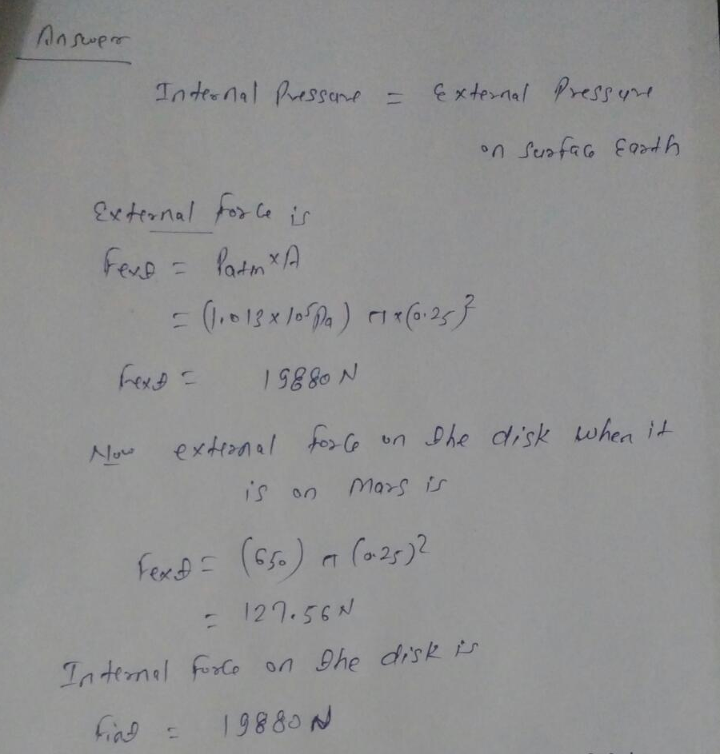Therefore

The Net force on the disk is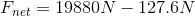which gives us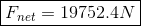or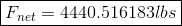or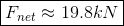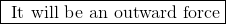Know the answer?
Your Answer:

#### Post as a guest

Your Name:

What's your source?

#### Earn Coin

Coins can be redeemed for fabulous gifts.

Not the answer you're looking for? Ask your own homework help question. Our experts will answer your question WITHIN MINUTES for Free.
Similar Homework Help Questions
• ### Suppose that the hatch on the side of a Mars lander is built and tested on Earth so that the internal pressure just bala...

Suppose that the hatch on the side of a Mars lander is built and tested on Earth so that the internal pressure just balances the external pressure. The hatch is a disk 50.0 cm in diameter. When the lander goes to Mars, where the external pressure is 650 N/m^2, what will be the net force (in newtowns and pounds) on the hatch, assuming that the internal pressure is the same in both cases? Will it be an inward or outward...

• ### Hydrology question 4. (20 pts) Curling is clearly the most exciting sport on Earth. Let's pretend...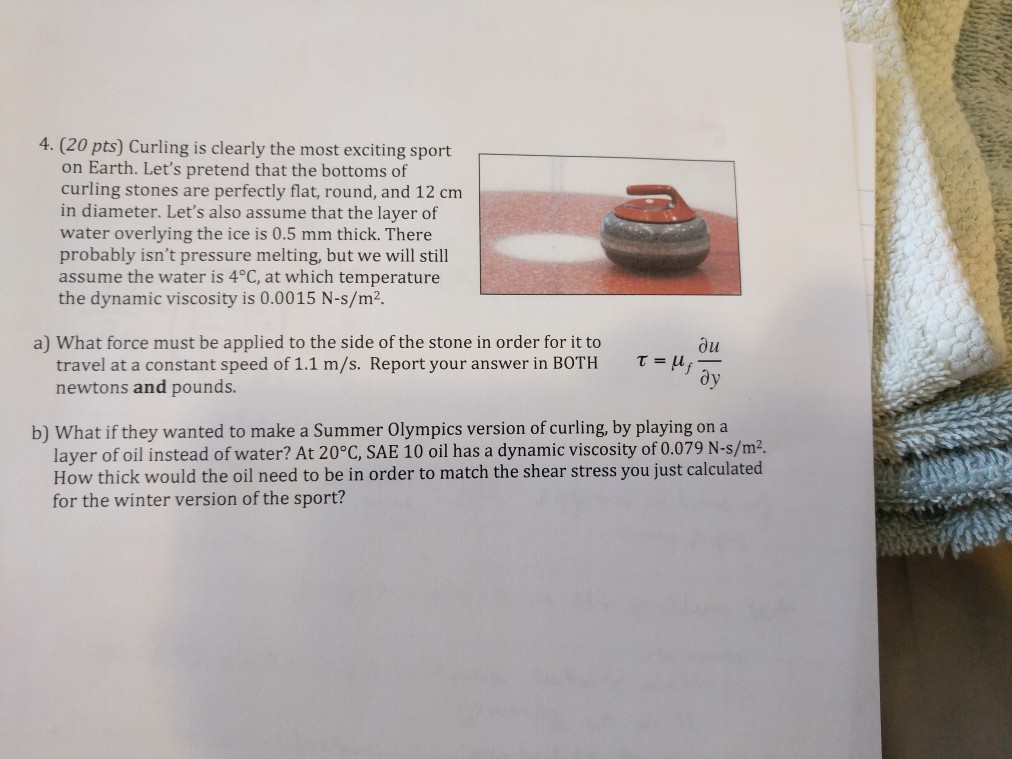Hydrology question 4. (20 pts) Curling is clearly the most exciting sport on Earth. Let's pretend that the bottoms of curling stones are perfectly flat, round, and 12 cm in diameter. Let's also assume that the layer of water overlying the ice is 0.5 mm thick. There probably isn't pressure melting, but we will still assume the water is 4°C, at which temperature the dynamic viscosity is 0.0015 N-s/m2. a) What force must be applied to the side of the...

Free Homework App

Scan Your Homework
to Get Instant Free Answers
Need Online Homework Help?

Get Answers For Free
Most questions answered within 3 hours.